# Logical Circuits

Logical Gates

A logic gate is an elementary building block of a digital circuit. Most logic gates have two inputs and one output. At any given moment, every terminal is in one of the two binary conditions low (0) or high (1), represented by different voltage levels. The logic state of a terminal can, and generally does, change often, as the circuit processes data. In most logic gates, the low state is approximately zero volts (0 V), while the high state is approximately five volts positive (+5 V).

There are seven basic logic gates: AND, OR, XOR, NOT, NAND, NOR, and XNOR.

The AND gate is so named because, if 0 is called "false" and 1 is called "true," the gate acts in the same way as the logical "and" operator. The following illustration and table show the circuit symbol and logic combinations for an AND gate. (In the symbol, the input terminals are at left and the output terminal is at right.) The output is "true" when both inputs are "true." Otherwise, the output is "false."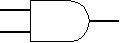AND gate

 Input 1 Input 2 Output 1 1 1 1

The OR gate gets its name from the fact that it behaves after the fashion of the logical inclusive "or." The output is "true" if either or both of the inputs are "true." If both inputs are "false," then the output is "false."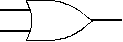OR gate

 Input 1 Input 2 Output 1 1 1 1 1 1

The XOR ( exclusive-OR ) gate acts in the same way as the logical "either/or." The output is "true" if either, but not both, of the inputs are "true." The output is "false" if both inputs are "false" or if both inputs are "true." Another way of looking at this circuit is to observe that the output is 1 if the inputs are different, but 0 if the inputs are the same.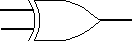XOR gate

 Input 1 Input 2 Output 1 1 1 1 1 1

A logical inverter , sometimes called a NOT gate to differentiate it from other types of electronic inverter devices, has only one input. It reverses the logic state.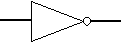Inverter or NOT gate

 Input Output 1 1

The NAND gate operates as an AND gate followed by a NOT gate. It acts in the manner of the logical operation "and" followed by negation. The output is "false" if both inputs are "true." Otherwise, the output is "true."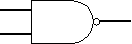NAND gate

 Input 1 Input 2 Output 1 1 1 1 1 1 1

The NOR gate is a combination OR gate followed by an inverter. Its output is "true" if both inputs are "false." Otherwise, the output is "false."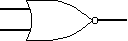NOR gate

 Input 1 Input 2 Output 1 1 1 1 1

The XNOR (exclusive-NOR) gate is a combination XOR gate followed by an inverter. Its output is "true" if the inputs are the same, and"false" if the inputs are different.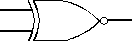XNOR gate

 Input 1 Input 2 Output 1 1 1 1 1 1

Using combinations of logic gates, complex operations can be performed. In theory, there is no limit to the number of gates that can be arrayed together in a single device. But in practice, there is a limit to the number of gates that can be packed into a given physical space. Arrays of logic gates are found in digital integrated circuits (ICs). As IC technology advances, the required physical volume for each individual logic gate decreases and digital devices of the same or smaller size become capable of performing ever-more-complicated operations at ever-increasing speeds.

Logic Circuits, Boolean Algebra, and Truth Tables

Logic Representation
There are three common ways in which to represent logic.
1.  Truth Tables
2. Logic Circuit Diagram
3. Boolean Expression
We will discuss each herein and demonstrate ways to convert between them.

Truth Tables
A truth table is a chart of 1s and 0s arranged to indicate the results (or outputs) of all possible inputs.  The list of all possible inputs are arranged in columns on the left and the resulting outputs are listed in columns on the right.  There are 2 to the power n possible states (or combination of inputs).  For example with three inputs there are 2^3=8 possible combination of inputs.Logic Diagram

A logic diagram uses the pictoral description of logic gates in combination to represent a logic expression.  An example below shows a logic diagram with three inputs (A, B, and C) and one output (Y).  The interpretation of this will become clear in the following sections.Boolean Expression

Boolean Algebra can be used to write a logic expression in equation form.  There are a few symbols that you’ll recognize but need to redefine.Note: Sometimes when the ! is used to represent the NOT it is used before the letter and sometimes it is used after the letter.  Care should be used so that you understand which method is being used!
Below is an example boolean expression.  In fact, it represents the same logic as the example logic circuit diagram above.Converting from a Logic Circuit Diagram to a Truth Table

This conversion is accomplished by selecting each state (or combination of inputs) one at a time, replacing the inputs with their respective values and figuring the value of each point through the circuit until the output is reached.   The final output value for each state is then listed in the truth table next to the value of each input.

Below is a logic circuit diagram with the input values.  Study it carefully for an extended period of time, it is an animated image and the inputs and output will change every few seconds.Below are the results of the conversion in truth table formConverting Logic Circuit Diagrams to Boolean Expressions

To convert from a logic circuit diagram to a boolean expression we start by listing our inputs at the correct place and process the inputs through the gates, one gate at a time, writing the result at each gate’s output.  The following is the resulting boolean expression of each of the gates.And here is an example of the process being carried out.  The fact that the result simplifies to the XOR is merely coincidental.Converting Truth Tables to Boolean Expressions

There are two methods for converting truth tables to boolean expressions.

The Sum of ProductsThe Product of SumsConverting Boolean Expressions to Logic Diagrams

Converting boolean expressions to logic diagrams is the most challenging conversion on this page because it requires a very good understanding of order of operation.  Below is the order of operations used in this conversion.Converting a Truth Table to a Logic Diagram

The easiest way to accomplish this is to first convert the truth table to a boolean expression and then to a logic diagram.